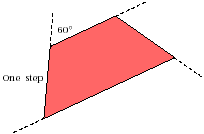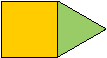www.MathEd.page/angles

Adapted from Geometry Labs, by Henri Picciotto

# Walking Convex Polygons

Equipment: Pattern Blocks, Unlined Paper

Definitions:
A polygon is a closed figure with straight sides.
An n-gon is a polygon with n sides.
A convex polygon is one where no angle is greater than 180°.
1. Is there such a thing as a nonconvex triangle? If so, sketch one; if not, explain why not.

2. Is there such a thing as a nonconvex quadrilateral? Sketch one, or explain why there's no such thing.

3. For each number of sides from 3 to 12, make a convex pattern block polygon. Use your template to record your solutions on a separate sheet.

In this activity, you will give each other instructions for walking polygons. As an example, here are instructions for walking the pattern block trapezoid. We will use a scale of one step for one inch:

 Walk forward one step from the starting point; Turn right 60°; Walk forward one step; Turn right 60°; Walk forward one step; Turn right 120°; Walk forward two steps; Turn right 120°.The final turn is not necessary, since the whole perimeter has been walked by then. However, it is traditional to include it, because it brings the walker back exactly to the starting position.

1. Mark the remaining steps and angles on the figure.

Note: The turn angle is also called the exterior angle. It is not usually the same as the interior angle, which is usually called simply the angle.

1. Write another set of instructions for walking the trapezoid, starting at another vertex.

2. Write instructions for walking each of the other pattern blocks.

3. Take turns with a partner, following each other's instructions for walking different blocks.

4. Make a two-block convex pattern block polygon, such as the one in the figure, and write instructions for walking it.## Discussion

1. Write alternate instructions for walking the trapezoid with one or more of these additional constraints:
• Walking backward instead of forward
• Making left turns instead of right turns
• Starting in the middle of the long side
1. What is the relationship between the turn angle and the interior angle? When are they equal?

2. How can you tell whether a polygon walk is clockwise or counterclockwise by just reading the walk's instructions?